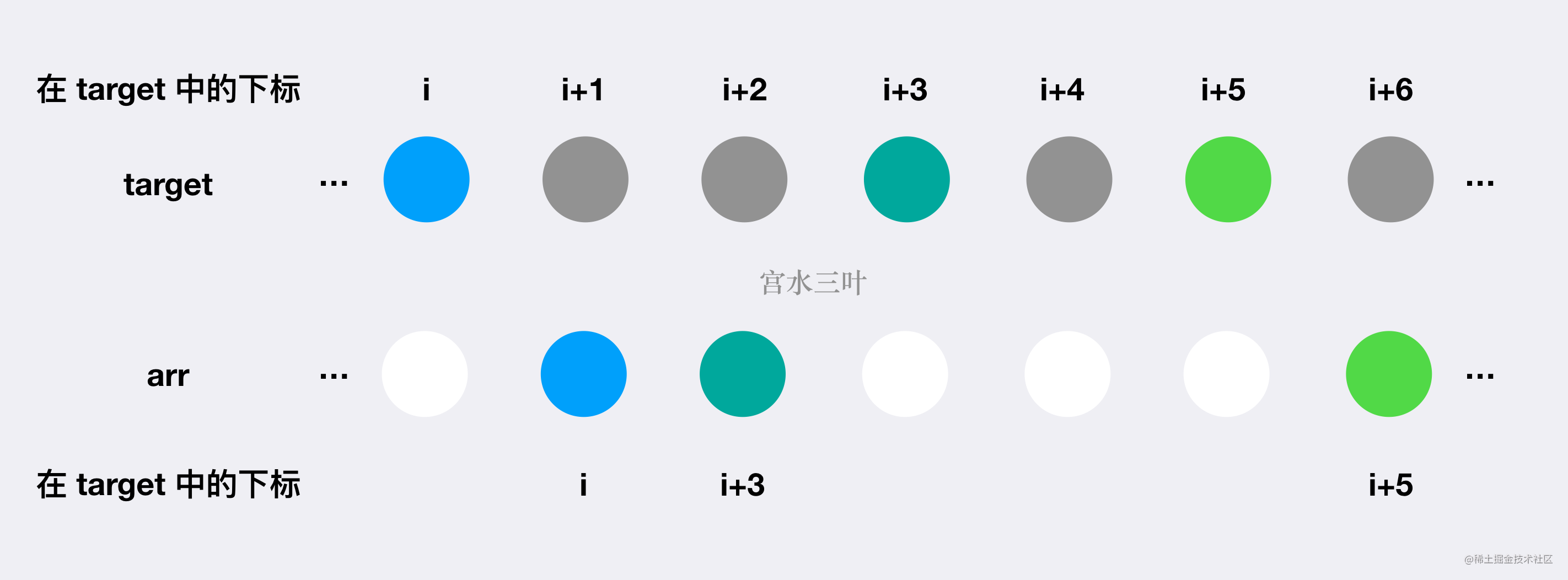# 【每日算法】详解为何能从 LCS 问题转化为 LIS 问题，以及 LIS 贪心解的正确性证明 ｜Python 主题月

## 题目描述

Tag : 「最长公共子序列」、「最长上升子序列」、「贪心」、「二分」

输入：target = [5,1,3], arr = [9,4,2,3,4]

输入：target = [6,4,8,1,3,2], arr = [4,7,6,2,3,8,6,1]

• 1 <= target.length, arr.length <= $10^5$
• 1 <= target[i], arr[i] <= $10^9$
• target 不包含任何重复元素。

## 证明

#### 1. 为何其中一个数组元素各不相同，LCS 问题可以转换为 LIS 问题？#### 2. 贪心求解 LIS 问题的正确性证明？

LIS 的贪心解法则是维护一个额外 $g$ 数组，$g[len] = x$ 代表上升子序列长度为 $len$ 的上升子序列的「最小结尾元素」为 $x$

• $f$ 动规数组：与朴素 LIS 解法的动规数组含义一致。$f[i]$ 代表以 $nums[i]$ 为结尾的上升子序列的最大长度；
• $g$ 贪心数组：$g[len] = x$ 代表上升子序列长度为 $len$ 的上升子序列的「最小结尾元素」为 $x$

• $g[i] = g[j] = x$：这意味着某个值 $x$ 既能作为长度 $i$ 的上升子序列的最后一位，也能作为长度为 $j$ 的上升子序列的最后一位。 根据我们对 $g$ 数组的定义，$g[i] = x$ 意味在所有长度为 $i$ 上升子序列中「最小结尾元素」为 $x$，但同时由于 $g[j] = x$，而且「上升子序列」必然是「严格单调」，因此我们可以通过删除长度为 $j$ 的子序列后面的元素（调整出一个长度为 $i$ 的子序列）来找到一个比 $g[i]$ 小的合法值。 也就是我们找到了一个长度为 $i$ 的上升子序列，且最后一位元素必然严格小于 $x$。因此 $g[i] = g[j] = x$ 恒不成立；

• $g[i] > g[j] = x$：同理，如果存在一个长度为 $j$ 的合法上升子序列的「最小结尾元素」为 $x$ 的话，那么必然能够找到一个比 $x$ 小的值来更新 $g[i]$。即 $g[i] > g[j]$ 恒不成立。

## 动态规划 + 贪心 + 二分

Java 代码：

class Solution {
public int minOperations(int[] t, int[] arr) {
int n = t.length, m = arr.length;
Map<Integer, Integer> map = new HashMap<>();
for (int i = 0; i < n; i++) {
map.put(t[i], i);
}
List<Integer> list = new ArrayList<>();
for (int i = 0; i < m; i++) {
int x = arr[i];
}
int len = list.size();
int[] f = new int[len], g = new int[len + 1];
Arrays.fill(g, Integer.MAX_VALUE);
int max = 0;
for (int i = 0; i < len; i++) {
int l = 0, r = len;
while (l < r) {
int mid = l + r + 1 >> 1;
if (g[mid] < list.get(i)) l = mid;
else r = mid - 1;
}
int clen = r + 1;
f[i] = clen;
g[clen] = Math.min(g[clen], list.get(i));
max = Math.max(max, clen);
}
return n - max;
}
}

Python 3 代码：

class Solution:
def minOperations(self, t: List[int], arr: List[int]) -> int:
n, m = len(t), len(arr)
map = {num:i for i,num in enumerate(t)}
lt = []
for i in range(m):
x = arr[i]
if x in map:
lt.append(map[x])
length = len(lt)
f, g =  * length, [inf] * (length + 1)
maximum = 0
for i in range(length):
l, r = 0, length
while l < r:
mid = l + r + 1 >> 1
if g[mid] < lt[i]:
l = mid
else:
r = mid - 1
clen = r + 1
f[i] = clen
g[clen] = min(g[clen], lt[i])
maximum = max(maximum, clen)
return n - maximum

• 时间复杂度：通过 $O(n)$ 复杂度得到 $target$ 的下标映射关系；通过 $O(m)$ 复杂度得到映射数组 $list$；贪心求解 LIS 的复杂度为 $O(m\log{m})$。整体复杂度为 $O(n + m\log{m})$
• 空间复杂度：$O(n + m)$